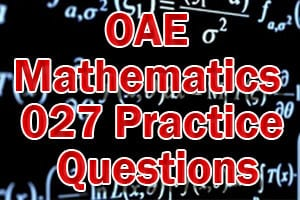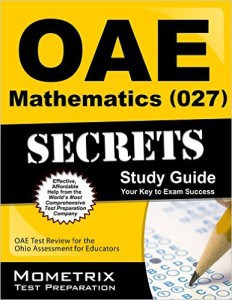# OAE Mathematics 027 Practice Questions

The OAE Mathematics 027 Exam is a computer based, 150 multiple choice question exam, which allows candidates to demonstrate proficiency with the necessary math knowledge to become a state licensed math educator in Ohio. The exam is about four hours and 15 minutes long, and is available by appointment year round. The exam questions are divided into five domains including (I) Math Processes and Number Sense, (II) Patterns, Algebra, and Functions, (III) Measurement and Geometry, (IV) Trigonometry and Calculus, and (V) Statistics, Probability, and Discrete Mathematics.